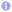Feature Engineering for Structured Data (numerical and categorical)

“Best Ingredients make Best Dish “, the same way “Best Features make Best Model”

As part of any Machine Learning project, does not matter whether it is related to Supervised Learning (i.e classification, Regression), or Unsupervised learning, best features make best model. The acquired data for the project may have numerous features, but in majority of cases, I am sure that all of them may not contribute enough to make best prediction. Hence, it is the responsibility of a Machine Learning Engineer / Statistical Modeler to understand and finalize the best features for the model.

“Feature Engineering” is a thought process, this is what can be a differentiator between a matured data science engineer, and a beginner. A matured data scientist, would always spends enough time to analyze the data to find best features for the model where he/she needs domain expertise on the problem he/she is solving, needs expertise on statistical analysis methods like correlation, co-variance, multi-collinearity, Hypothesis Testing, OLS (ordinary least square), Decision Tree or RandomForest model.

Usually we perform “Feature engineering” during Exploratory Data Analysis (EDA). Feature Engineering mainly involves two techniques,

• Feature selection
• Feature Extraction

Feature selection can be performed manually using statistical methods like Backward selection, Forward selection, Mixed Selection. Also, we can depend on Decision Tree or Random Forest Model to find the best features automatically.

The below is the code snippet, that performs backward selection (Please reach us info@datajango.com, in case you demand the code explanation)

 Source code```def buildModel(df_train_set, numerical_features):
"""Builds OLS Model, we can find the significant features here based on p-values"""
formula_input_features = '+'.join(numerical_features)
multi_reg_model = smf.ols(formula='SalePrice ~ ' + formula_input_features, \
data=df_train_set).fit()
return multi_reg_model

def backWordSelection(df_train_set, numerical_features):
"""This method automates the manual feature selection, here the feature selection \
happens based on p-value, lower the p-value (less than 0.05), greater the \
predictive power"""
count = 0 #number of models built
for i in range(len(numerical_features[:])):
multi_reg_model = buildModel(df_train_set, numerical_features)
count = count+1 #increment by 1 when model is built
sorted_pvalues = multi_reg_model.pvalues.sort_values(ascending=False)
sorted_pvalues.drop('Intercept', inplace=True)
if(sorted_pvalues > 0.05):
drop_feature = sorted_pvalues.index.values
sorted_pvalues.drop(drop_feature, inplace=True)
numerical_features = sorted_pvalues.index.values
#print ("\n",len(numerical_features),"\n")
else:
break
return count,numerical_features, multi_reg_model```

The below is the code snippet, that make use of RandomForest model and gives us the best features in descending order. As shown below, top few best features can be seen whenever you call an attribute called “feature importance” on the RandormForest model.

 Source code```digit_dataset = pd.read_csv('digit_recognizer_train.csv')
digit_X = digit_dataset.iloc[:, 1:]
digit_y = digit_dataset['label']
digit_X_train, digit_X_test, digit_y_train, digit_y_test = \
train_test_split(digit_X, digit_y, test_size=0.2)

rnd_clf = RandomForestClassifier(random_state=42)
rnd_clf.fit(digit_X_train, digit_y_train)

# use rnd_clf.feature_importances_
for feature, imp_score in sorted(zip(digit_dataset.columns, rnd_clf.feature_importances_), key=lambda x: x, reverse=True):
if(imp_score > 0.0001): #you can take any appropriate value here
print(feature, imp_score)```

L1 Regularization technique  (Lasso Regression) would also give us a clue on finding best features. When L1 Regularization  is applied over data, it may result with “0” coefficients for certain features, meaning is those features are not significant/important.You can use ElasticNet Regression as well.

 Source code```for (fet, coef) in zip(X_features, elst_model.coef_):
if coef == 0:
print(fet, coef)

OutPut:
BsmtQual 0.0
BsmtExposure 0.0
BsmtFinType1 0.0
BsmtFinType2 0.0
GarageType 0.0
GarageFinish 0.0
GarageQual 0.0
GarageCond 0.0
Neighborhood_Names 0.0```

On other hand, Feature Extraction is a way where we create new feature(s) based on existing features. Best example is the unsupervised technique called “dimensionality reduction”, example is PCA (Principle Component Analysis). This technique should be only used whenever you have plenty of features (hundreds and thousands of features) in input space. In other cases, with some simple mathematical calculations or data manipulation techniques, you may derive new features. Example is, if you have a feature called “Name” where you have the values like “Mr.Coppolla”, “Ms.Kate” etc, you can derive a new feature called as “Gender” feature based on “Name” feature such a way that when you find a prefix “Mr” in Name column, you generate a value called “Male” in corresponding Gender feature.

The above narrated steps can help you to pick the best features for your model, so that you can have a best model for your Machine Learning problem.

By
— Praveen Vasari

Categories: